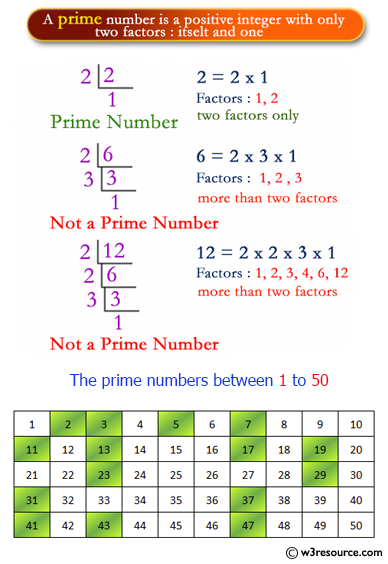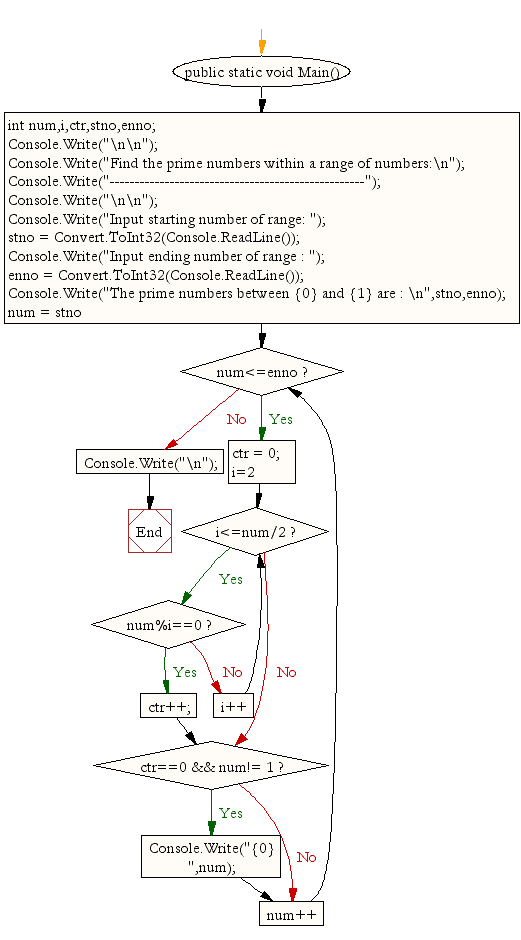﻿ C# - Find the prime numbers within a range of numbers# C# Sharp Exercises: Find the prime numbers within a range of numbers

## C# Sharp For Loop: Exercise-34 with Solution

Write a program in C# Sharp to find the prime numbers within a range of numbers.

Pictorial Presentation:Sample Solution:

C# Sharp Code:

``````using System;
public class Exercise34
{
public static void Main()
{
int num,i,ctr,stno,enno;

Console.Write("\n\n");
Console.Write("Find the prime numbers within a range of numbers:\n");
Console.Write("---------------------------------------------------");
Console.Write("\n\n");

Console.Write("Input starting number of range: ");
Console.Write("Input ending number of range : ");
Console.Write("The prime numbers between {0} and {1} are : \n",stno,enno);

for(num = stno;num<=enno;num++)
{
ctr = 0;

for(i=2;i<=num/2;i++)
{
if(num%i==0){
ctr++;
break;
}
}

if(ctr==0 && num!= 1)
Console.Write("{0} ",num);
}
Console.Write("\n");
}
}
```
```

Sample Output:

```Find the prime numbers within a range of numbers:
---------------------------------------------------
Input starting number of range: 1
Input ending number of range : 50
The prime numbers between 1 and 50 are :
2 3 5 7 11 13 17 19 23 29 31 37 41 43 47
```

Flowchart:C# Sharp Code Editor:

Contribute your code and comments through Disqus.

What is the difficulty level of this exercise?

Test your Programming skills with w3resource's quiz.

﻿